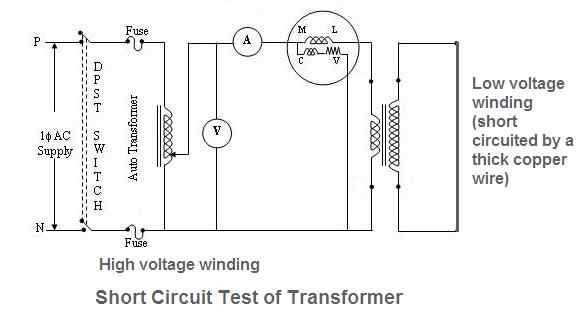# Short Circuit Test of TransformerThe set up for short circuit test of transformer is shown in the figure. An auto transformer is used in the circuit to adjust the input voltage precisely to the rated voltage.

The ammeter, voltmeter and watt meter are connected in the circuit to measure the current, voltage and power respectively.

In this test generally, the high voltage side is connected to the AC supply and the low voltage side is short circuited with the help of thick copper wire.

## Procedure for Short Circuit Test of Transformer

• Connect the circuit as shown in the figure.
• Keep the auto transformer output at its minimum voltage position and switch on the AC supply.
• Increase the applied voltage very slowly, and adjust it to get the current equal to the rated value of the winding. Do not increase the applied voltage further.
• Note down the watt meter, voltmeter and ammeter readings. Let these are Wsc, Vsc and Isc

## Parameter Calculations

The short circuit test on transformer is performed at the primary and secondary rated currents. Therefore the total copper loss is the full load copper loss.

As the iron losses are supply voltage dependent and supply voltage in this test is small, the iron losses will be negligibly small.

Hence the reading (Wsc) shown by the wattmeter is almost entirely corresponding to the full load copper loss.

We know that Wsc = VscIsccos φsc
or cos φsc = Wsc / (VscIsc)

Therefore, Wsc = Copper loss = I2scR01
or R01 = Wsc / I2sc

Where R01 is the total equivalent resistance of the transformer referred to the primary.

Similarly Z01 = Vsc / Isc = (R201 + X201)1/2

or X01 = (Z201 – R201)1/2

Where X01 is the total equivalent reactance of the transformer referred to primary.

In this way parameters R01, X01, Z01 and full load copper losses can be calculated from the short circuit test on transformer.

If the transformation ratio K is known, it is possible to obtain these parameters referred to the secondary side.

## Efficiency Calculation from Short Circuit Test of Transformer

Example: A 250/500V transformer gave the following test results:

• Short circuit test with low voltage winding short circuited; 20V, 12A 100W.
• Open circuit test on low voltage side; 250V, 1A, 80W.

Determine the efficiency of the transformer when the output is 10A, 500V at 0.8 p.f. lagging.

Solution: From the short circuit test (on H.V. side)

In the short circuit test of transformer, the wattmeter measures the copper losses of the transformer on short circuit secondary current(I2sc).

Output secondary current, I2 = 10A
Copper losses at this current, Pc = (I2/I2sc)2Wc = (10/12)2 x 100 = 69.44W

From open circuit test:
In the open circuit test of transformer, the wattmeter measures the iron losses occurred in the transformer.
Therefore, iron losses in the transformer (Pi) = 80W

Output power at given load = V2I2cosɸ2 = 500 x 10 x 0.8 = 4000W

Efficiency of transformer = [output/(output + Pi + Pc)] x 100
= [4000/(4000 + 80 + 69.44)] x 100 = 96.4%

Transformer | All Posts

© https://yourelectricalguide.com/ short circuit test of transformer.

### 3 thoughts on “Short Circuit Test of Transformer”

1.Copper loss at load current 10 A in HV winding should be [(20/12)2 x 100 ] A = 277.78 A ; because 10 A in HV winding corresponds to 20 A in LV winding.

1.Correction :
Copper loss at load current 10 A in HV winding should be [(20/12)2 x 100 ] W = 277.78 W

2.Correction in my previous comment:
Copper loss should be [(20/12)2 x 100 ] W = 277.78 W